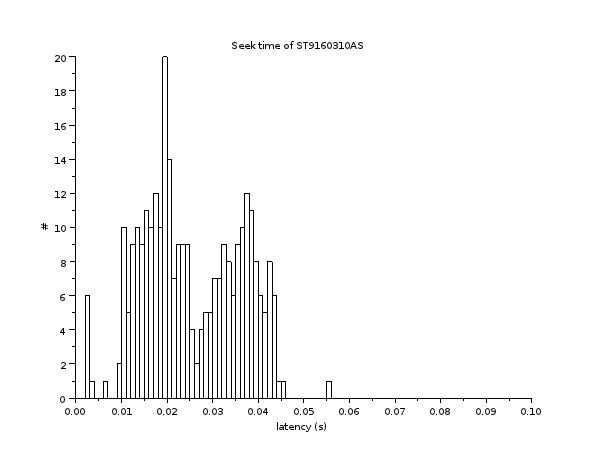# Precisely measuring the maximal disk latency

by Martin Monperrus

The maximal end-to-end latency of a hard-drive is achieved with accessing a random sector (it includes transfer time). The program seeker.c published at http://www.linuxinsight.com/how_fast_is_your_disk.html exactly measures this latency and calls it random access time. This post presents a shell program that does the same thing as seeker.c but in a more flexible way. In particular, it allows setting the block size, hence to simulate reading a whole file system block and it outputs all intermediary seek times, in order to compute the median, standard deviation and to display histograms. Note that the measured latency is slightly higher than real disk latency due to the time of launching and completing dd. With no parallelization at the disk level (i.e. a queue_depth of 1), the number of seeks per second is equal to 1/latency.

To use it: `\$ ./seeker.sh /dev/sda`.

To compute the metrics and histogram using scilab, first collect the data with `\$ ./seeker.sh /dev/sda 300 > latency.dat`
Then a scilab program can be:
```latency = fscanfMat('latency.dat');
median(latency)
mean(latency)
stdev(latency)
histplot(0:0.001:0.1,latency,normalization=%f)
axes=gca()
axes.x_label.text = 'latency (s)'
axes.y_label.text = '#'
xtitle('Seek time')
```
Example output:Note that the latency measures given by bonnie++ are very misleading, because they are an average of latency under heavy read/write, which means when the disk is completely saturated. Both the averaging and the saturation yield very high values (the latency distribution then contains many outliers).

The program:
```#!/bin/bash
# Measures the end-to-end maximal latency of a hard drive (which includes seek time, a.k.a. random access time)
# Usage: ./seeker.sh /dev/sda
# Inspired from seeker.c at http://www.linuxinsight.com/how_fast_is_your_disk.html
# See http://www.monperrus.net/martin/precisely+measuring+the+maximal+disk+latency

if [[ ! -z \$1 ]]
then
F=\$1
else
F=/dev/sda
fi

if [[ ! -z \$2 ]]
then
N=\$2
else
N=10
fi

DISKSIZE=`blockdev --getsize64 \$F`
echo disk size: \$DISKSIZE bytes >&2

# ext3 block size: 4096
BLOCKSIZE=4096
echo block size: \$BLOCKSIZE >&2

MAXSEEK=\$((DISKSIZE/BLOCKSIZE))
echo max dd seek index: \$MAXSEEK >&2

# bash constant of \$RANDOM
RANDOM_MAX=32767

TIMEFORMAT="%E"

for i in `seq 1 \$N`
do
SEEK=\$(((RANDOM*MAXSEEK)/RANDOM_MAX))
A=`time dd if=\$F of=/dev/null ibs=\$BLOCKSIZE skip=\$SEEK count=1 2>&1`
done 2>&1

```
Tagged as: